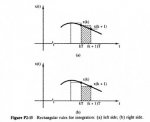# Help understand Left and Right Rules

#### XaviPacheco

##### New member
The rectangular rules for numerical integration are illustred in Figure P2-15. The left side rule is despicted in Figure P2-15a, and the right side rule is despicted in Figure P2-15b. The integral of x(t) is approximated by the sum of the rectangular areas shown for each rule. Let y(kT) be the numerical integral x(t), 0<=t<=kT.

Write de difference equation relating y(k+1), y(k), and x(k) for the left side.
Write de difference equation relating y(k+1), y(k), and x(k+1) for the right side.

I know that the answers are:

a) For the left side:
y(k+1) = y(k) +Tx(k)

a) For the right side:
y(k+1) = y(k) +Tx(k+1)

I really don't understand clearly why is it. I'm reading about left and right side rules, but when it comes to do this exercise, I find it confused. Can anyone explain it to me in simple words?#### tkhunny

##### Moderator
Staff member
It's all about the height of the little rectangles. How tall shall we draw them? There are only two choices, right and left.

Say, your first rectangle is sitting on the x-axis taking up space between 1/2 and 3/4. f(x) is your function. Here is your choice. What will be the height of the rectangle you draw on [1/2,3/4]? W know it's 1/4 wide, but how tall is it?

If we pick f(1/2), we have chosen the left rule. Remember that it's a rectangle, so the other vertical side is the same height.
If we pick f(3/4), we have chosen the right rule. Remember that it's a rectangle, so the other vertical side is the same height.

In a later lesson, there will be other choices.

#### sinx

##### Junior Member
integral helpi did not learn with left and right side rules, but i will try to explain.
with this graph, the left side rule gives you a rectangle too big, the right side rule gives you one too small. When the rectangle's horizontal side shrinks to zero (with integration), both left and right side rules are the same; i.e. you have a sliver of the rectangle. Then the curve is the top of the rectangle (the section of the curve is so small it is a straight line).
Imagine finding the area under the curve by using a straight edge and pencil, and draw a series of straight vertical lines under the curve, so close together they made a black box. Adding (the areas of) all the pencil lines is finding the area by integration.

#### stapel

##### Super Moderator
Staff member
It's all about the height of the little rectangles. How tall shall we draw them? There are only two choices, right and left.
If we use sub-interval endpoints, yes, there are only the two choices. But there's something called "The Midpoint Rule" that suggests other options....